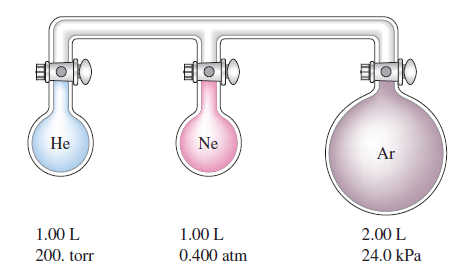Chemistry Practice Problems Partial Pressure Practice Problems Solution: Consider the three flasks in the diagram below. As...

🤓 Based on our data, we think this question is relevant for Professor Ratliff's class at USF.

# Solution: Consider the three flasks in the diagram below. Assuming the connecting tubes have negligible volume, what is the partial pressure of each gas and the total pressure after all the stopcocks are opened?

###### Problem

Consider the three flasks in the diagram below. Assuming the connecting tubes have negligible volume, what is the partial pressure of each gas and the total pressure after all the stopcocks are opened?###### Solution

Concept: Boyle's Law

From the Ideal Gas Law we get Boyle's Law.        P1V1 = P2V2

Notice that V2 is the total volume after all the stopcocks are opened.         V2 =  1 L + 1 L + 2 L = 4 L

Now we need to find the partial pressures of each gas separately.View Complete Written Solution

Partial Pressure

Partial Pressure

#### Q. A 1.00-L gas sample at 100.°C and 600. torr contains 50.0% helium and 50.0% xenon by mass. What are the partial pressures of the individual gases?

Solved • Thu Oct 18 2018 12:39:45 GMT-0400 (EDT)

Partial Pressure

#### Q. A tank contains a mixture of 52.5 g oxygen gas and 65.1 g carbon dioxide gas at 27°C. The total pressure in the tank is 9.21 atm. Calculate the partia...

Solved • Thu Oct 18 2018 10:06:51 GMT-0400 (EDT)

Partial Pressure

#### Q. Consider the flask apparatus below, which now contains 2.00 L H  2 at a pressure of 360. torr and 1.00 L N2 at an unknown pressure. If the total press...

Solved • Thu Oct 18 2018 09:52:33 GMT-0400 (EDT)

Partial Pressure

#### Q. Consider the flasks in the following diagram. What are the final partial pressures of H 2 and N2 after the stopcock between the two flasks is opened? ...

Solved • Thu Oct 18 2018 09:49:19 GMT-0400 (EDT)